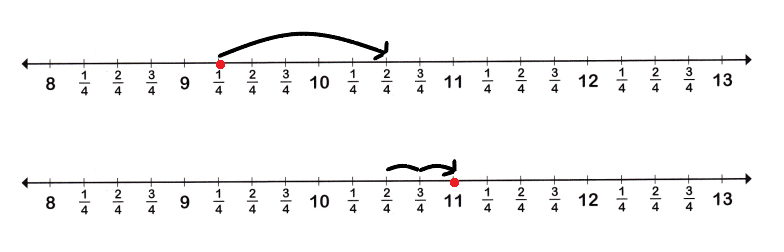# McGraw Hill Math Grade 4 Chapter 11 Lesson 8 Answer Key Problem Solving: Using a Number Line

Practice the questions of McGraw Hill Math Grade 4 Answer Key PDF Chapter 11 Lesson 8 Problem Solving: Using a Number Line to secure good marks & knowledge in the exams.

## McGraw-Hill Math Grade 4 Answer Key Chapter 11 Lesson 8 Problem Solving: Using a Number Line

Solve

Use the number line to add or subtract.Question 1.
Laith has a book that is 9$$\frac{1}{4}$$ in. long. Jason’s book is 1 $$\frac{2}{4}$$ in. longer than Laith’s. How long is Jason’s book?Explanation:
Laith has a book that is 9$$\frac{1}{4}$$ in. long
Jason’s book is 1 $$\frac{2}{4}$$ in. longer than Laith’s
I marked the numbers on the number line and drew jumps forward to add
9$$\frac{1}{4}$$ in. + 1$$\frac{2}{4}$$ in. = 11
So, Jason’s book is 11 in.

Question 2.
Mia has a string that is 11$$\frac{3}{4}$$ in. long. Ian has a string that is 2$$\frac{2}{4}$$ in. shorter than Mia’s. How long is Ian’s string?Mia has a string that is 11$$\frac{3}{4}$$ in. long
Ian has a string that is 2$$\frac{2}{4}$$ in. shorter than Mia’s
11$$\frac{3}{4}$$ in. – 2$$\frac{2}{4}$$ in. = 9$$\frac{1}{4}$$ in.
So, Lan’s string 9$$\frac{1}{4}$$ in. long.